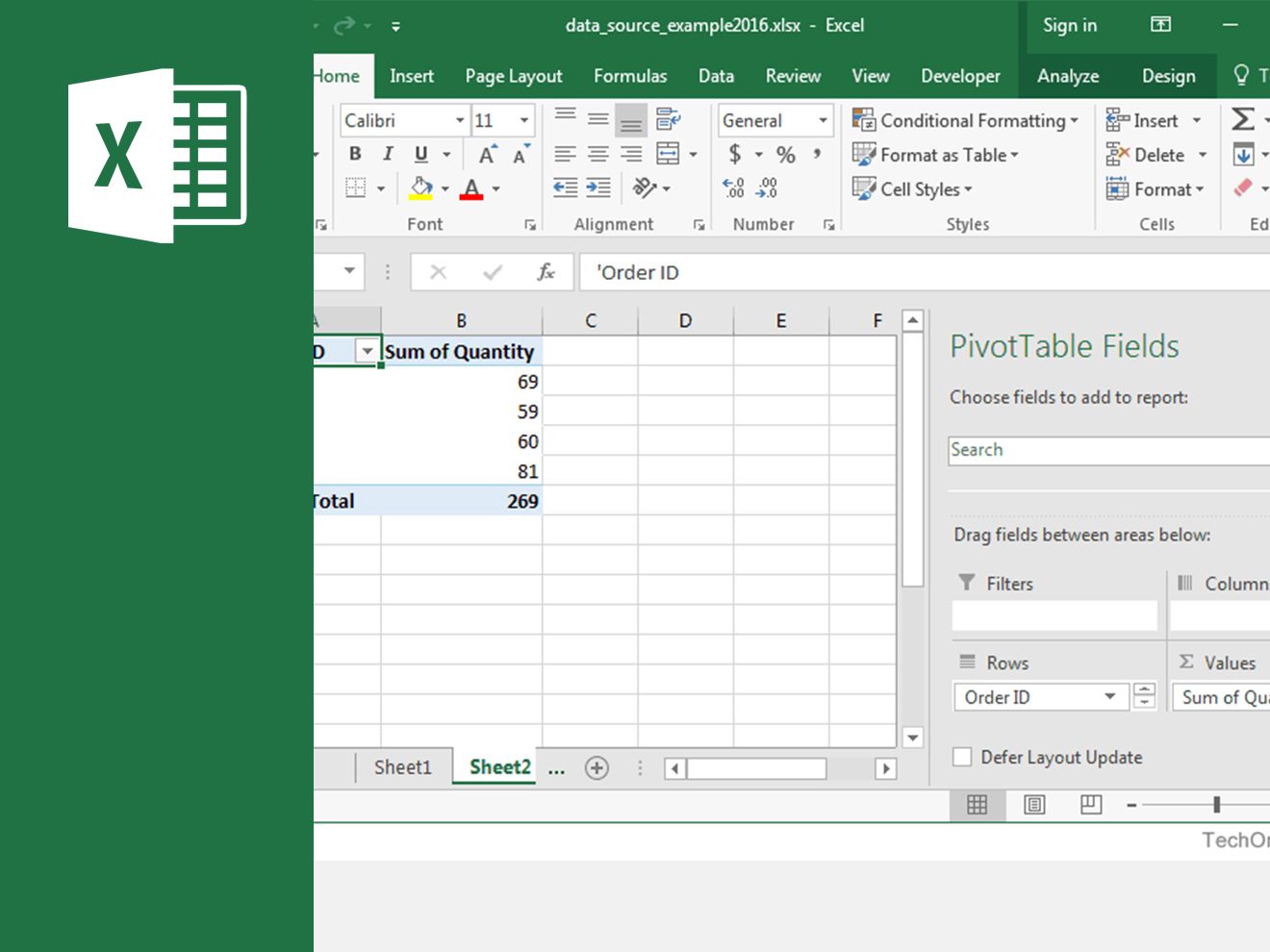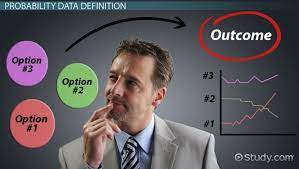I thought I knew. LOL (Laugh out Loud). Coming into Data Analytics class, I thought it would be easy sailing when our Lecturer Dr. Francis Okoye brought up excel on the board. But alas, I was wrong.

This is not to say I was a novice but I definitely was no expert. The list of keyboard shortcuts alone, left me bewildered.

But in all it was interesting relearning what I thought I knew.

A. What is Excel?

Microsoft Excel is powerful data visualization and analysis software, which uses spreadsheets to store, organize, and track data sets with formulas and functions. Excel is used by marketers, accountants, data analysts, and other professionals.

I was able to learn the following and more;

1. Creating a new spreadsheet from scratch.
2. Executing basic computations like adding, subtracting, multiplying, and dividing.
3. Writing and formatting column text and titles.
4. Using Excel’s auto-fill features.
6. Keeping column and row titles visible as you scroll past them in a spreadsheet, so that you know what data you’re filling as you move further down the document.
7. Sorting your data in alphabetical order.
8. Excel has 17,179,869,184 cells
9. Excel has 1,048,576 rows
10. Excel has 16,384 columns
11. There should be no empty rows
12. There should be no empty columns
13. No hidden cells
14. One row for all headers

B. Excel Formulas

In Excel, you can use the following formulas to carry out some complex functions — without adding to the complexity.

1. Equal sign: Before creating any formula, you’ll need to write an equal sign (=) in the cell where you want the result to appear.
2. Addition: To add the values of two or more cells, use the + sign. Example: =C5+D3.
3. Subtraction: To subtract the values of two or more cells, use the  sign. Example: =C5-D3.
4. Multiplication: To multiply the values of two or more cells, use the * sign. Example: =C5*D3.
5. Division: To divide the values of two or more cells, use the sign. Example: =C5/D3.
6. You can create a formula that adds, subtracts, multiplies, and divides all in one cell. Example: =(C5-D3)/((A5+B6)*3).

C. Excel Functions

Excel functions are automated formulas you would use in a task. For example, instead of using the + sign to add up a range of cells, you’d use the SUM function.

Some examples of Excel functions include:

1. SUM: The SUM function automatically adds up a range of cells or numbers. To complete a sum, you would input the starting cell and the final cell with a colon in between. Example: =SUM(C5:C30).
1. AVERAGE: The AVERAGE function averages out the values of a range of cells. Example: =AVERAGE(C5:C30).
1. IF: The IF function allows you to return value based on a logical test. Example: =IF(A2>B2,”Over Budget”,”OK”).

D. Documents created with Excel.

These are a few of the documents that can be created using Excel:

1. Income Statements
2. Balance Sheets
3. Calendar

References:

#MEMBA11## Next Generation Fixed Wireless Access (NGFWA) for Service Providers: A Viable Alternative to Traditional Fiber-Optic Networks

in## Transforming Nigeria’s Local Government System for Rural Economic Growth and Increased GDP

in